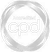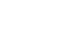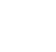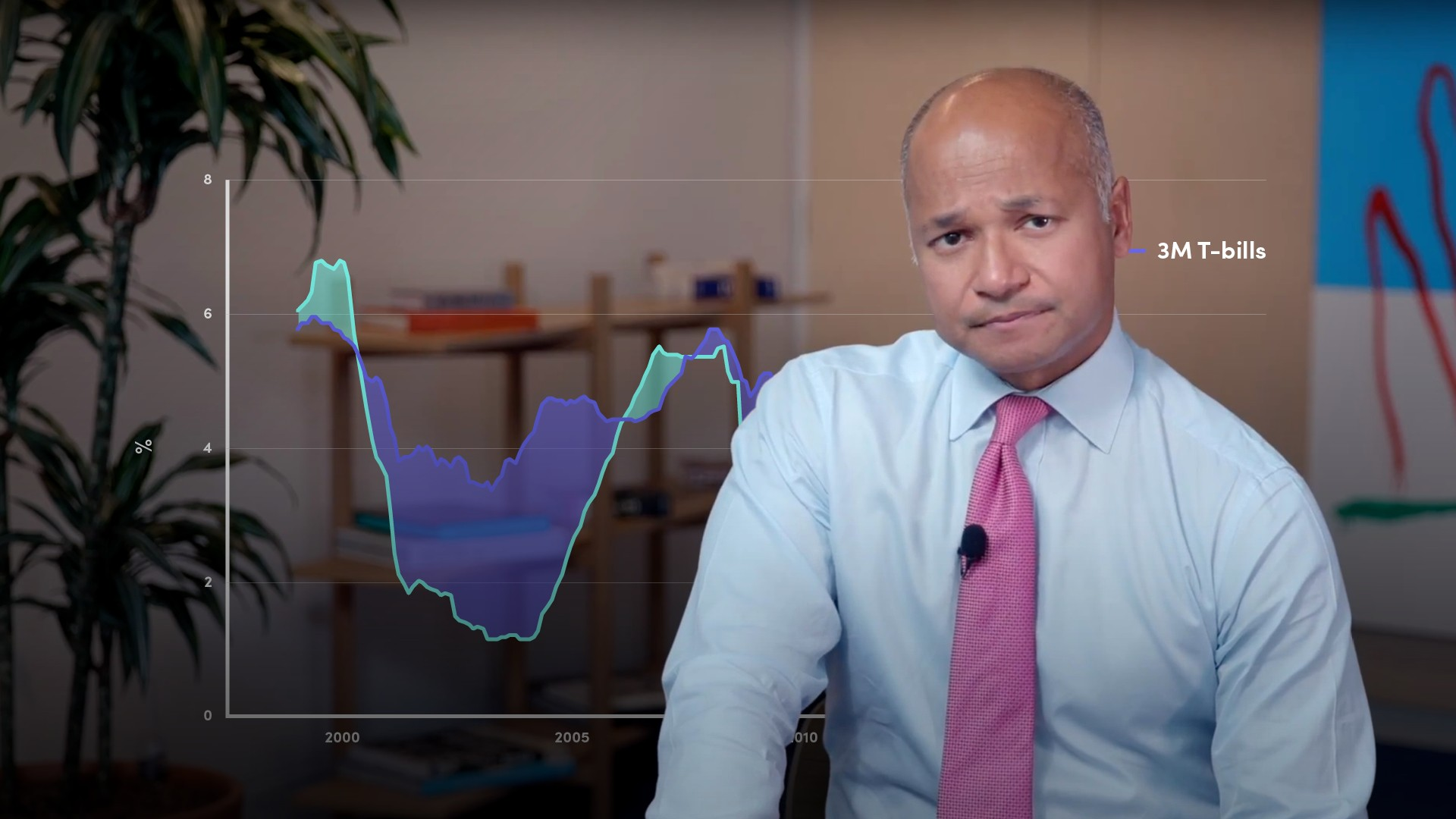# Interest Rate Risk in the Banking Book Introduction25 years: Treasury & ALM

For a bank, the act of undertaking ordinary customer business generates interest rate risk. Moorad explains: why this is the case, the different forms of interest rate risk, and strategies used to manage these risks.

Treasury Risk ManagementFor a bank, the act of undertaking ordinary customer business generates interest rate risk. Moorad explains: why this is the case, the different forms of interest rate risk, and strategies used to manage these risks.

Finance Unlocked is the video learning platform built for finance professionals.### Interest Rate Risk in the Banking Book Introduction

11 mins 47 secs

Overview

The banking book is where conventional bank loan and deposit products are held without repricing and the majority of banks operate solely with a banking book. Due to fluctuations in market interest rates there is interest rate risk inherent in the banking book, this is known as Interest Rate Risk in the Banking Book (IRRBB). When interest rates change, the timing and present value of future cash flows change, affecting the underlying value of a bank’s economic value. The forms of interest rate risk are: gap risk, optionality, basis risk, and yield curve risk. To manage these risks, banks use gap analysis to measure mismatches between assets and liabilities.

Key learning objectives:

• Define IRRBB

• Understand the forms of interest rate risk

• Gain an understanding of the Interest Rate Gap Report

## Book a demo to access

Summary

#### What is the banking book?

Conventional bank loan and deposit products that do not reprice while they are on the balance sheet, are held on the “banking book”, also referred to as an “accruals” book. This is an accounting term. What this means is that, when a customer loan or deposit is originated, its full notional value is recorded on the balance sheet at inception, and this notional value remains unchanged, other than for the impact of interest (either paid or received by the bank) for its entire life. The notional value of the loan or deposit is not reviewed every day and revalued.  Also, the asset or loan is expected to be held on the bank’s balance sheet for its entire contractual maturity. This is why the banking book is also known as the “hold-to-maturity” or HTM portfolio.

#### What is the gap interest rate risk?

Either one, or both of assets and liabilities, may be originated at a floating rate of interest, so the universal form of IRRBB that every bank is exposed to is what is known as “gap interest rate risk”, which is the risk that arises when the cost of the bank’s funding increases: there is a potential negative impact on net interest income. Also the origination of fixed-rate assets and liabilities gives rise to what one might term “accounting risk”, because if market rates rise or fall then there is a potential “opportunity cost foregone” impact and this will also be viewed negatively.  This explains the focus on IRRBB by the Basel committee and national regulators.

#### What is interest rate risk?

Interest rate risk in the banking book refers to the current or prospective risk to a bank’s earnings, and ultimately capital, arising from movements in interest rates. When interest rates change, the present value and timing of future cash flows change. This in turn affects the underlying value of a bank’s assets, liabilities and off-balance sheet instruments and hence its economic value. Excessive interest rate risk could pose a material threat to a bank’s current capital base and/or future earnings if not properly managed.

#### What are the forms of interest rate risk?

• Gap Risk - Assets (generally loans) do not match liabilities (funding) in terms of tenure, duration and currency. Where assets and liabilities are mismatched, adverse interest rate movements can lead to profitability benign reduced or eliminated
• Optionality - The bank may have match-funded a loan, but the client has an option to pre-pay that may be exercised. If rates fall, the matching assets need to be replaced at a lower rate than the original loan, which would reduce profitability or could result in a loss
• Yield Curve Risk - Rates may not change generally, but rather in only one part of the yield curve. In a short/lend long funding scenario, profitability is reduced if short-term rates increase
• Basis Risk - Assets and liabilities can be linked to floating rates of different benchmarks

#### How is risk measured?

The traditional method used is called a gap analysis, where assets and liabilities are each grouped together by maturity “buckets” (i.e. one day, one week, one month, two months, etc.). Banks estimate and use probable maturities for assets and liabilities with optionality (effective maturity as opposed to contractual maturity). Floating rate obligations are measured according to their next repricing date as opposed to their final maturity date.

#### What is the Interest Rate Gap Report?

A static interest rate gap report is used as a basis to assess gap risk, whether it is in relation to the effect of interest rate movements on income or economic value.

The report has separate columns for assets and liabilities and is divided into a number of distinct time buckets, ranging from 0-1 month, to a period normally in excess of 10 years. All cash items in the balance sheet (not accounting adjustments) are included in the report according to when they reprice, or, in the case of fixed rate items, when they mature.

#### What is the interest rate gap?

The difference between the assets and liabilities re-pricing in a given period is known as the interest rate gap. The cumulative gap captures the net position on all the assets and liabilities which re-price between 0 months and a given time bucket.

Within a given time bucket, a bank may have a positive, negative or neutral gap. The gap is positive when more assets reprice or mature than liabilities. In this case, the bank is said to be asset sensitive for the time band. An asset sensitive bank is generally expected to benefit from rising interest rates because its assets are expected to be re-priced more quickly than its liabilities. The converse is true for a negative gap, which occurs when more liabilities than assets mature in a given time bucket. A bank in this situation will be liability sensitive for the time band and expected to gain from falling rates (but lose from rising rates) as in this instance, its liabilities would reprice downwards more quickly than its assets.

## Book a demo to access

ExpertFormer senior banker with almost 30 years experience. Moorad has held senior roles in commerical, investment and retail banking. He has also spent the last five years as a visiting professor.09:58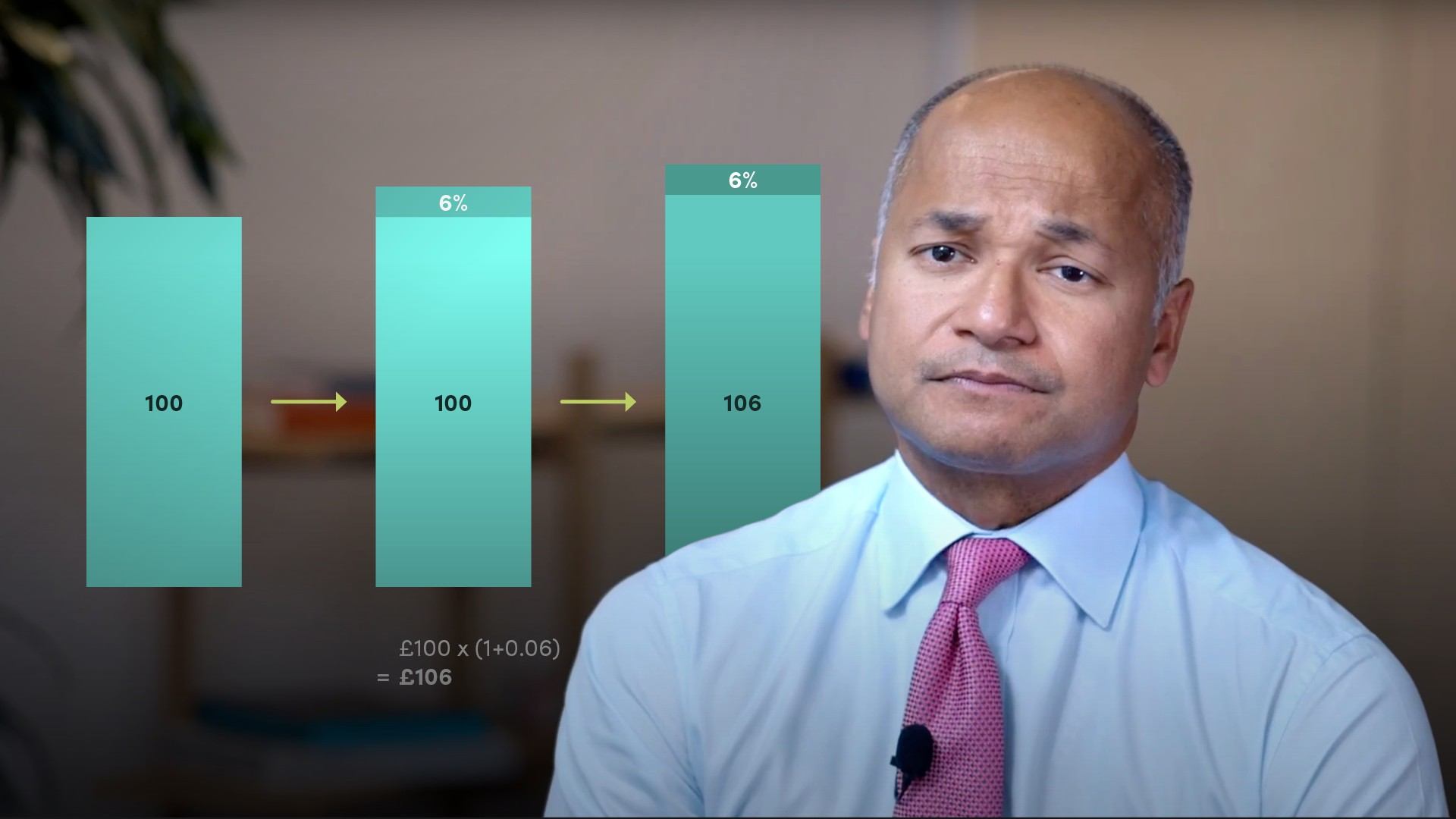09:4706:2605:2203:2614:5311:28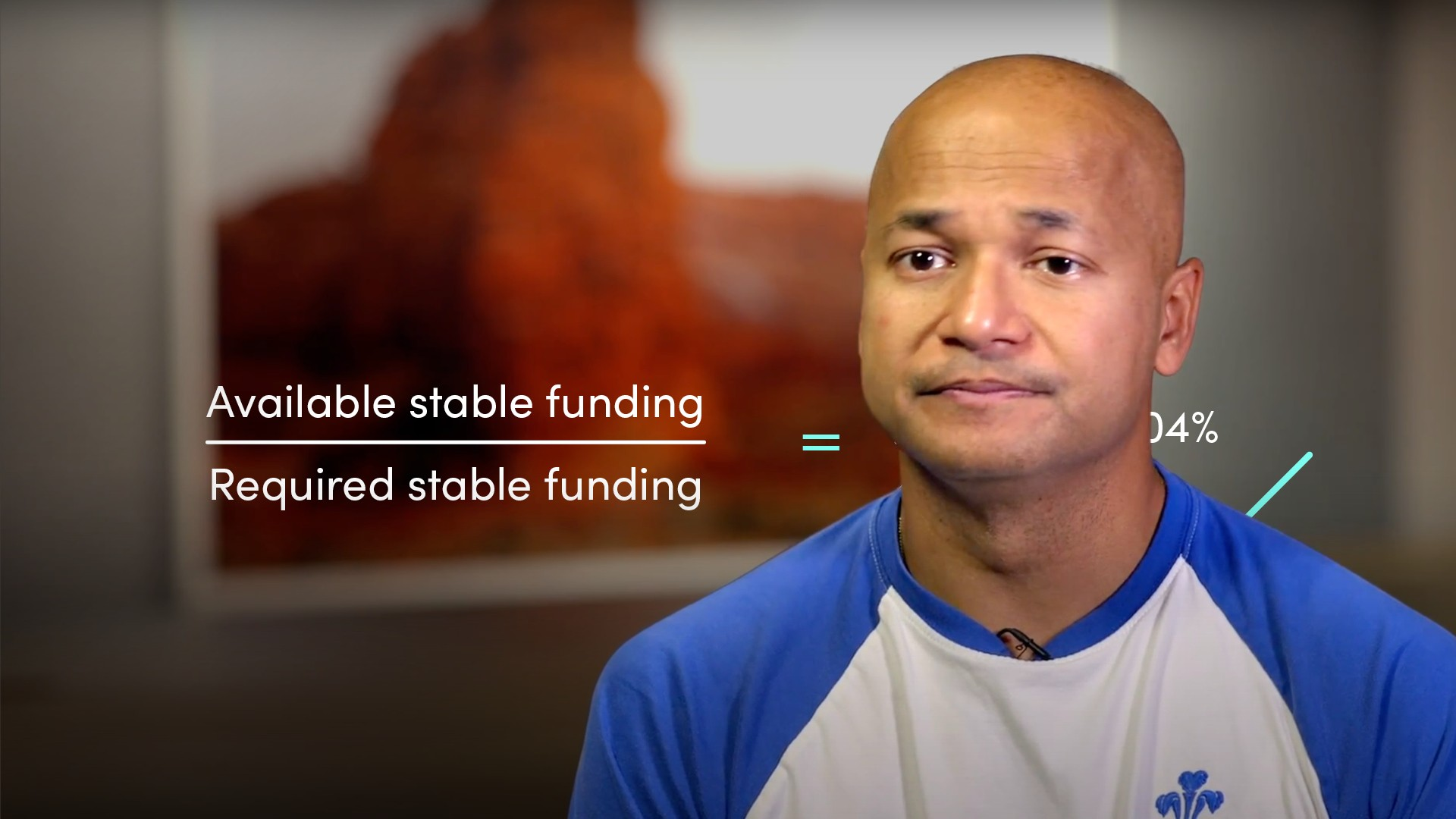07:3513:1915:25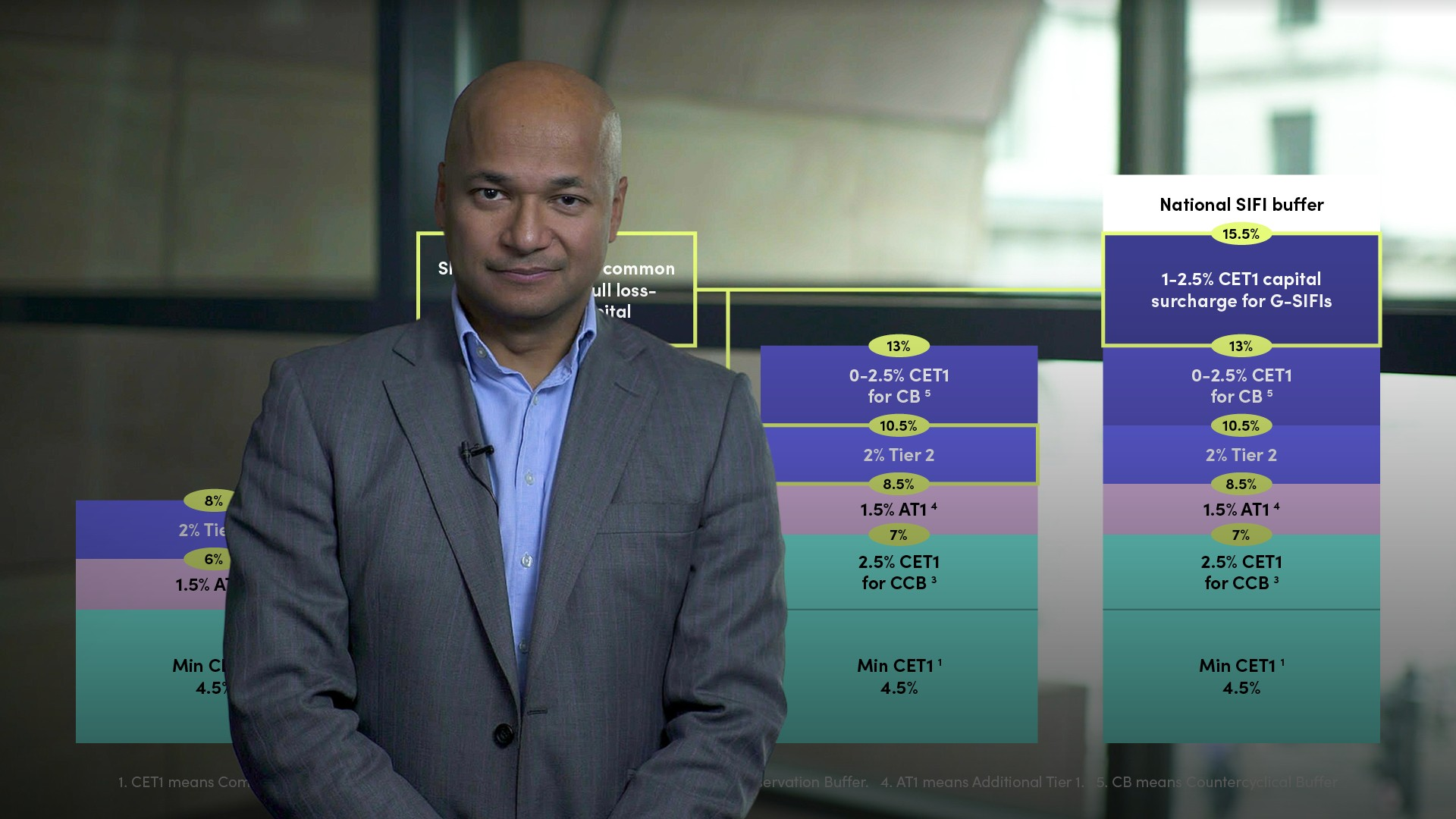11:0725:0919:44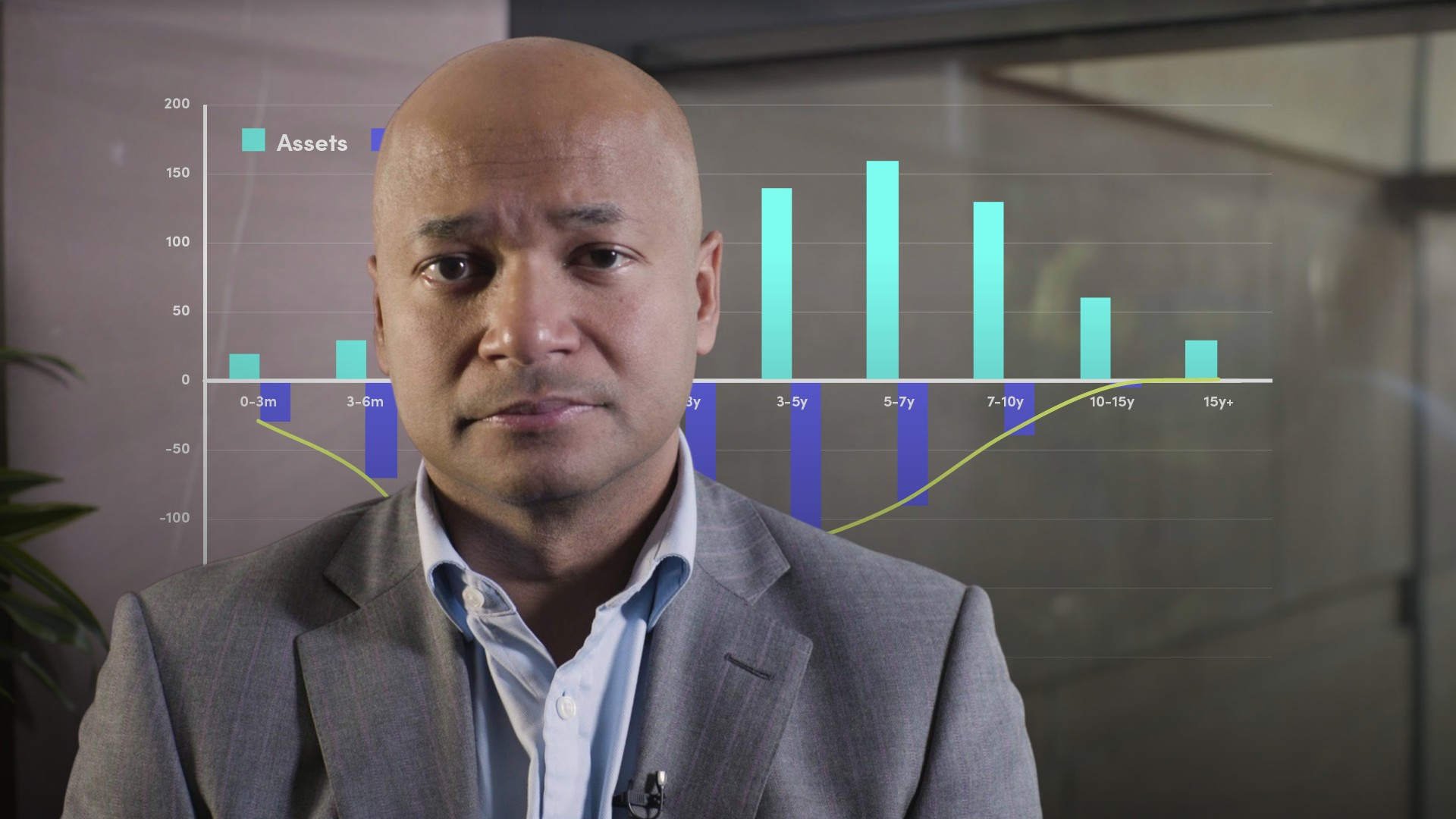17:04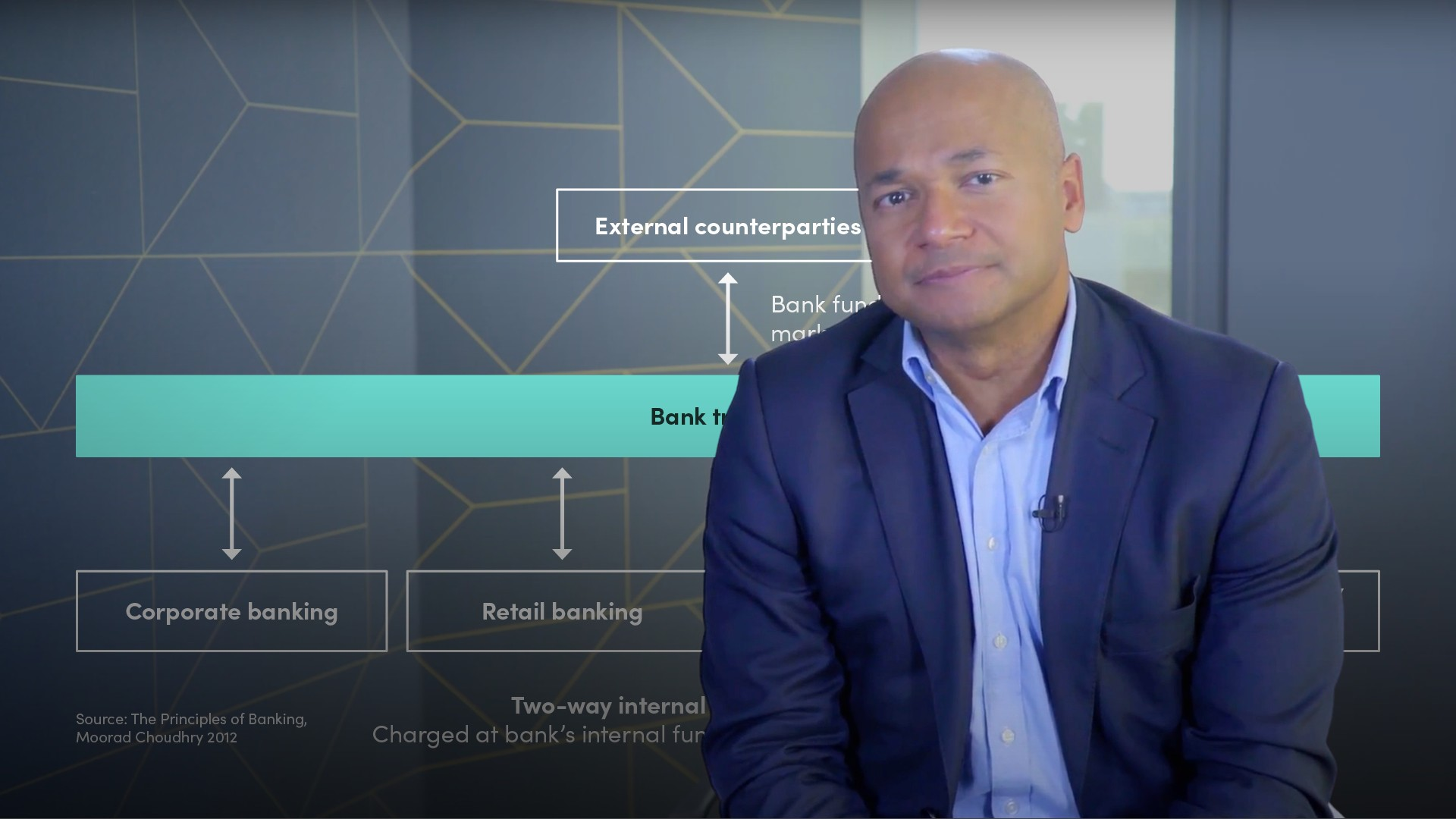16:5910:01

## Related videos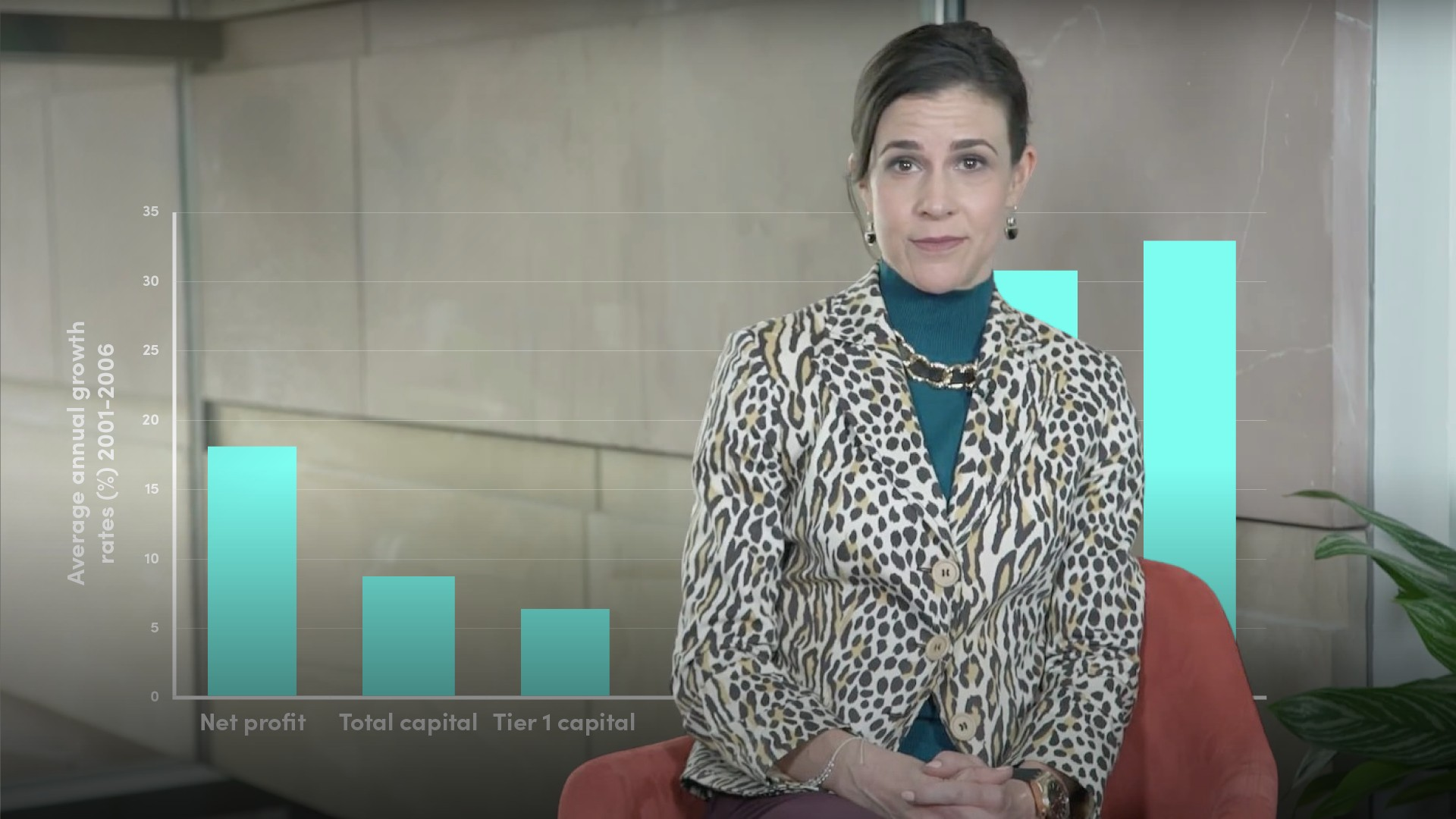Banking Essentials### Accredited by